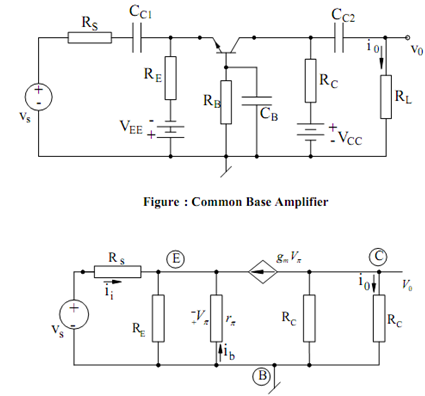## The Common-base Amplifier Assignment Help

Assignment Help: >> Transistor Amplifier Circuits - The Common-base Amplifier

Common-base Amplifier:

An exemplary common-base amplifier is illustrated in Figure and its small ac equivalent is illustrated in Figure. A routine analysis of the equivalent circuit gives :

V0  =- ( gm Vπ ) (Rc || RL )

Application of KCL at the emitter node gives :

g m  V π   + (Vπ / rπ )+ (Vπ / RE  )+ Vs  - (- Vπ ) /RS = 0

since β = gm rπ, we can write :

V π  ((1 +β  / r π )+ (1/RE  )+  (1/RS)) = -Vs/Rs

from where we get

Vπ =-      Vs  / Rs  [(rπ  /1 +β )|| RE  || Rs ]Figure: Small Signal Equivalent Circuit of Common Base Amplifier

The small signal voltage gain of the circuit is, thus, found to be

Av  = V0 /VS = + gm ((Rc || RL  )/ Rs) [(rπ/1+β || RE  || Rs )

as Rs → 0, Av  ≅ gm  (Rc || RL )

The current gain Ai =I0/Ii may be determined as follows. The KCL equation at the emitter is given by

I i  + (Vπ /r π )+ g m   v π + Vπ  /RE=0

from which we obtain

V π = - Ii =   (  rπ  /  ( 1+β) || RE]

The load current is given by

I0  =- ( gm Vπ ) (RC  / RC  + RL )

Hence,  I0 /Ii is found to be

Ai  =      I0  / Ii  = gm (Rc / Rc + RL) [[ rπ /1 + β)  || RE ]

In the limiting case, as RE → ∞ and RL → 0, then current gain becomes the short circuit current gain specified by

A i0   = gm rπ / 1 +β   =  β/1 +β    = α  (as expected)#### Assured A++ Grade

Get guaranteed satisfaction & time on delivery in every assignment order you paid with us! We ensure premium quality solution document along with free turntin report!

All rights reserved! Copyrights ©2019-2020 ExpertsMind IT Educational Pvt Ltd